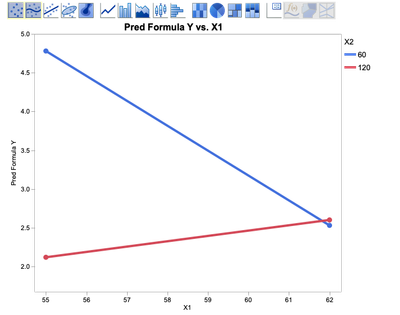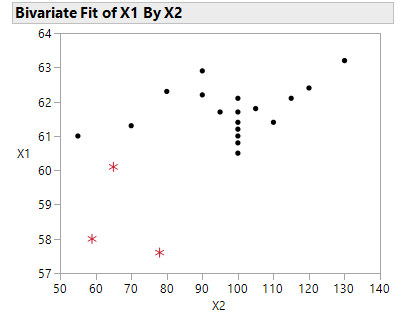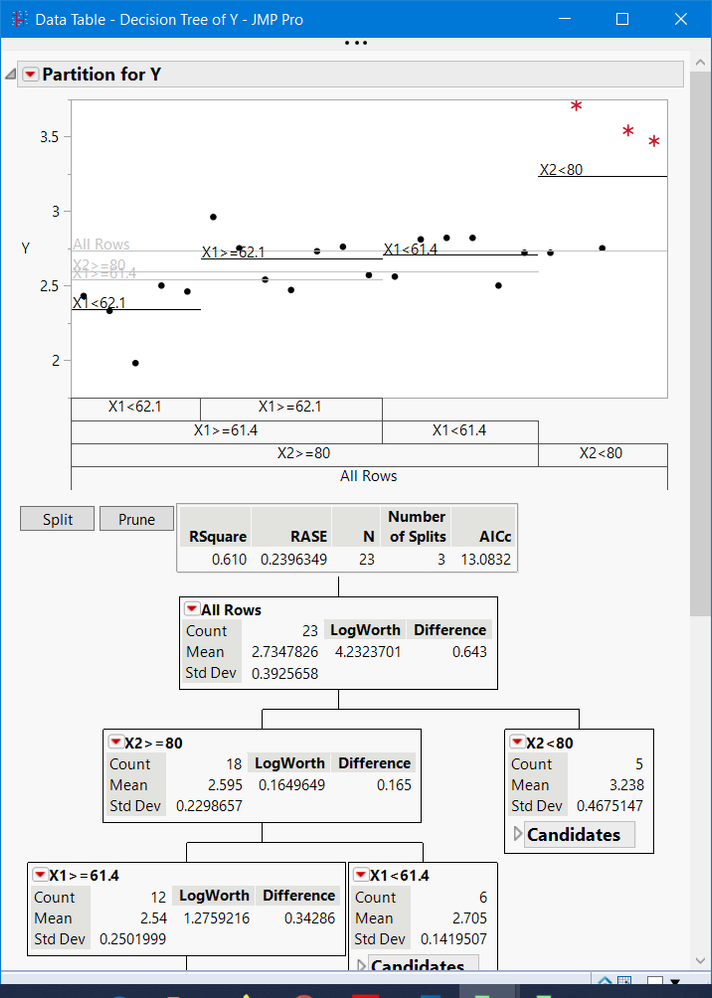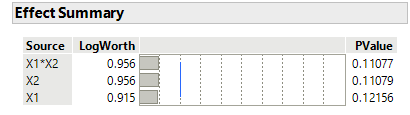Choose Language Hide Translation Bar
Highlighted

## How to get interaction plot for required factor values

Hi JMP Users,

I have data table with factor X1 and X2 and response Y. I am using JMP 14.3.0.

Based on least square linear fit, interaction term is significant.

Default interaction plot for the data is with X1 values 63.2 and 57.6, and for X2 55 and 130. (refer attachment).

I would need interaction plot with X1 value 55 and 62, and for X2 60 and 120.

Is there is way to represent the lines in interaction plot for above required values?

Thanks!

Attached: Data table and interaction plot

8 REPLIES 8
Highlighted

## Re: How to get interaction plot for required factor values

The only way that I know to change the interaction plot the way you want is to apply the Coding column property to the factor columns and set the low and high values to the range that you want. This way, though, also changes the estimates and interpretation of the model parameters.

Learn it once, use it forever!
Highlighted

Highlighted

## Re: How to get interaction plot for required factor values

You want something like this?Highlighted

## Re: How to get interaction plot for required factor values

I would actually love to see the similar values in interaction profiler using least square platform, but not at the cost of change in estimates for model parameters (one of the way, suggested by markbailey).
The suggested graph meet the purpose. Thank You!

I think for this graph, I would need to save prediction formula and use that formula on required input column X1 and X2 to get Y and then further plot data using graphs. Can you share\suggest navigation for the same. or Your navigation for the shared plot.
Thank You!!
Highlighted

## Re: How to get interaction plot for required factor values

@nil ,

The interaction term was significant, however, the data in the JMP table you provided, looks like happenstance data?? Below is the plot of your X1 and X2 factor levels(values). Following that is a partition of Y vs X1, X2 and X1*X2 (a calculated column)After removing the 3 extreme values at Low, Low, X1 and X2 values, a least squares fit Effect Summary is shown below.Your data indicates that X1 < 80 and X1 < 61 has some effect on Y (Y >3). I suggest you repeat these values or find additional data to support what this data set indicates.  In other words that this phenomenon is reproducible.

To get a defensible model, I recommend a planned experiment. Work with a statistician. If you are working for a company that does not have statistical help, you might want to contact a nearby university for help.

Good luck.

Highlighted

## Re: How to get interaction plot for required factor values

Hi gzmorgan0,
Thanks for your view on the data table. Suggestion are noted for further investigation.
Highlighted

## Re: How to get interaction plot for required factor values

Not sure why, but the new Fit Model Effects Summary was missing in my last post.  I edited it and added it back.

Less those 3 extreme points, your model has no significant effects.

Highlighted

## Re: How to get interaction plot for required factor values

hi gzmorgan0
that is quite possible. We are trying to see if additional data available.
thanks!
Article Labels